RS Aggarwal Class 7 Solutions Chapter 4 - Rational Numbers

RS Aggarwal Class 7 Chapter 4 - Rational Numbers Solutions Free PDF

A number that can be expressed as a fraction or a quotient of two integers p/q is called as a Rational number. Here, the numerator is ‘p’ and the denominator is ‘q’ and it has to be a non-zero denominator to fulfill the condition of a rational number. Two integers can also be represented as a ratio of two integers and a matrix which involves rational numbers can be termed as a rational matrix.

Some of the major arithmetic operations that can be performed with a rational number are:

2. Division
3. Multiplication
4. Subtraction
5. Inverse
6. Exponentiation
7. Equality of Rational Numbers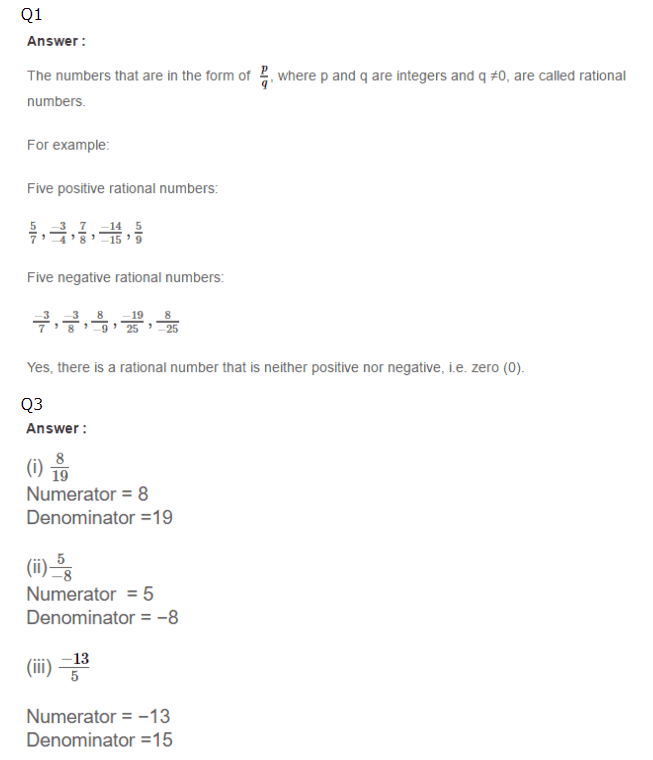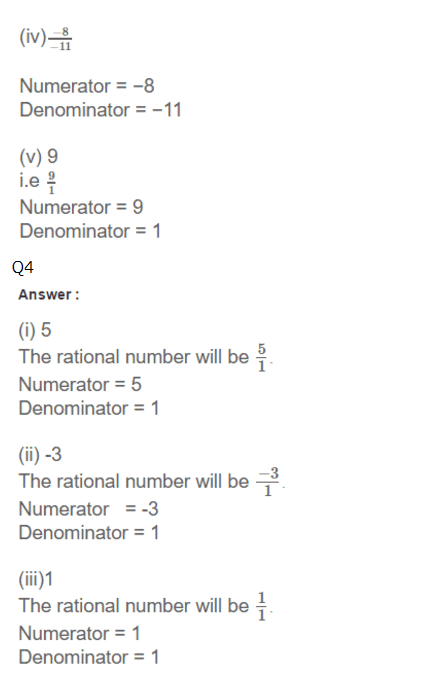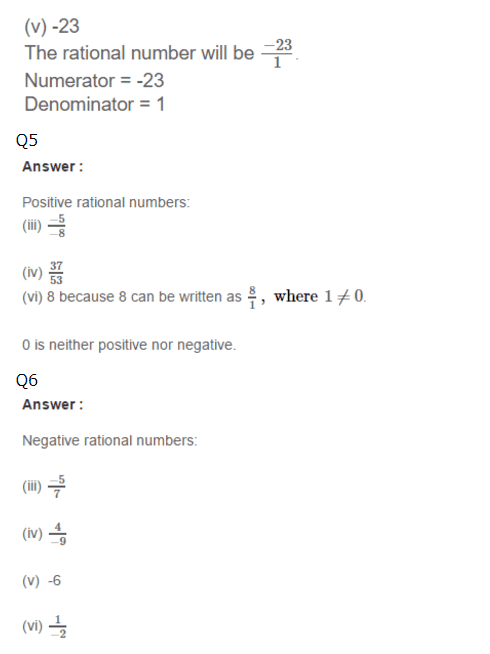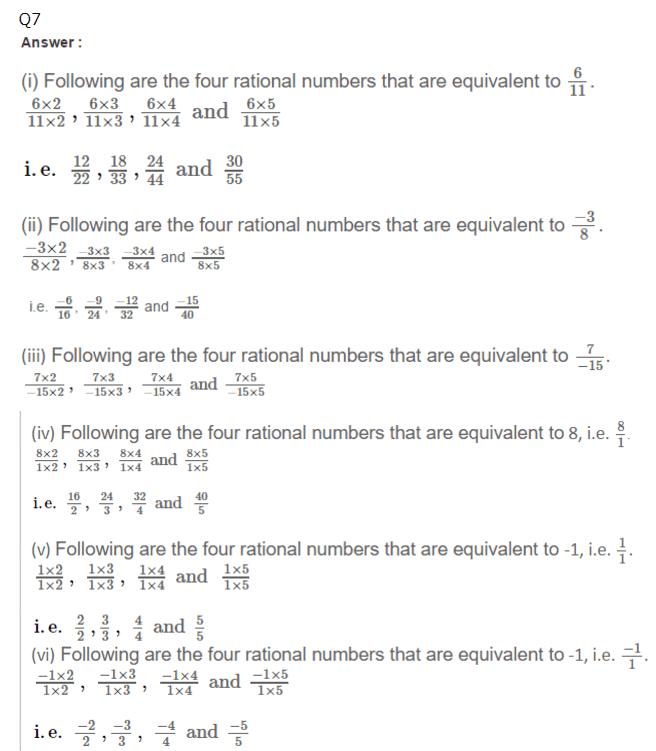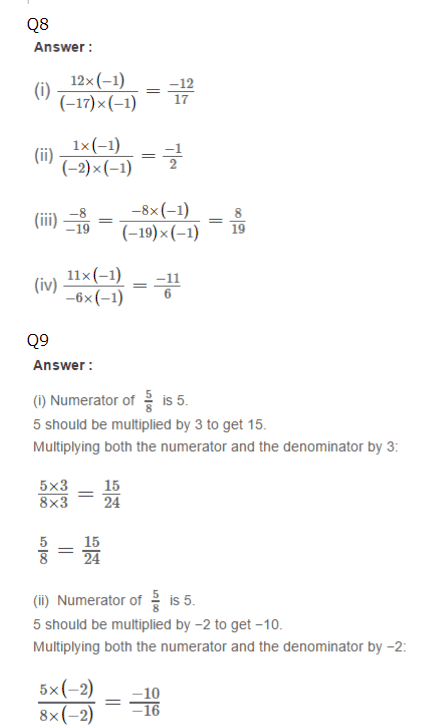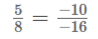Practise This Question

Factory waste is neutralised with an acid before disposing it into the water bodies.